### 2.2.2 Leptons

With the quarks and electron, we have met all the fundamental fermions required to make atoms, and almost all of the particles we need to discuss the Standard Model. Only one player remains to be introduced--the neutrino,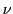. This particle completes the first generation of fundamental fermions:

 The First Generation of Fermions -- Charge Name Symbol Charge Neutrino0 Electron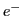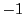Up quark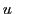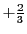Down quark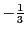Neutrinos are particles which show up in certain interactions, like the decay of a neutron into a proton, an electron, and an antineutrinoIndeed, neutrinoshave antiparticles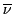, just like quarks and all other particles. The electron's antiparticle, denoted, was the first discovered, so it wound up subject to an inconsistent naming convention: the antielectron' is called a positron.

Neutrinos carry no charge and no color. They interact very weakly with other particles, so weakly that they were not observed until the 1950s, over 20 years after they were hypothesized by Pauli. Collectively, neutrinos and electrons, the fundamental fermions that do not feel the strong force, are called leptons.

In fact, the neutrino only interacts via the weak force. Like the electromagnetic force and the strong force, the weak force is a fundamental force, hypothesized to explain the decay of the neutron, and eventually required to explain other phenomena.

The weak force cares about the handedness' of particles. It seems that every particle that we have discussed comes in left- and right-handed varieties, which (quite roughly speaking) spin in opposite ways. There are are left-handed leptons, which we denote asand left-handed quarks, which we denote as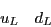and similarly for right-handed fermions, which we will denote with a subscript. As the terminology suggests, looking in a mirror interchanges left and right--in a mirror, the left-handed electron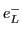looks like a right-handed electron,, and vice versa. More precisely, applying any of the reflections in the Poincaré group to the (infinite-dimensional) representation we use to describe these fermions interchanges left and right.

Remarkably, the weak force interacts only with left-handed particles and right-handed antiparticles. For example, when the neutron decays, we always haveand never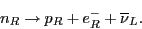This fact about the weak force, first noticed in the 1950s, left a deep impression on physicists. No other physical law is asymmetric in left and right. That is, no other physics, classical or quantum, looks different when viewed in a mirror. Why the weak force, and only the weak force, exhibits this behavior is a mystery.

Since neutrinos only feel the weak force, and the weak force only involves left-handed particles, the right-handed neutrinohas never been observed directly. For a long time, physicists believed this particle did not even exist, but recent observations of neutrino oscillations suggest otherwise. In this paper, we will assume there are right-handed neutrinos, but the reader should be aware that this is still open to some debate. In particular, even if they do exist, we know very little about them.

Note that isospin is not conserved in weak interactions. After all, we saw in the last section thatis all about counting the number ofquarks over the number ofquarks. In a weak process like neutron decaythe right-hand side has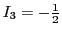, while the left has.

Yet maybe we are not being sophisticated enough. Perhaps isospin can be extended beyond quarks, and leptons can also carry. Indeed, if we defineand, we get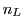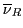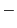where we have used the rule thatreverses sign for antiparticles.

This extension of isospin is called weak isospin since it extends the concept to weak interactions. Indeed, it turns out to be fundamental to the theory of weak interactions. Unlike regular isospin symmetry, which is only approximate, weak isospin symmetry turns out to be exact.

So from now on we shall discuss only weak isospin, and call it simply isospin. For thisequals zero for right-handed particles, and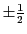for left-handed particles:

 The First Generation of Fermions -- Charge and Isospin Name Symbol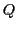Left-handed neutrino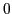Left-handed electronLeft-handed up quark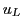Left-handed down quark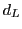Right-handed neutrinoRight-handed electronRight-handed up quarkRight-handed down quarkThe antiparticle of a left-handed particle is right-handed, and the antiparticle of a right-handed particle is left-handed. The isospins also change sign. For example,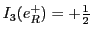, while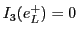.

In Section 2.3.2, we will see that the Gell-Mann-Nishijima formula, when applied to weak isospin, defines a fundamental quantity, the `weak hypercharge', that is vital to the Standard Model. But first, in Section 2.3.1, we discuss how to generalize the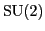symmetries from isospin to weak isospin.

2010-01-11